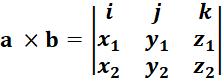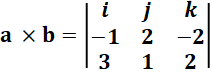# Cross Product Calculator

First vector (a)
i
j
k
Second vector (b)
i
j
k

## Right-hand rule in the cross product

Use our vector cross product calculator to calculate the cross product of two vectors. Specify the form in which both vectors are measured; by coordinates or by point. Enter the value of three variables X, Y, and Z for each vector in the given input boxes. If you choose the form of a vector by point, you have to enter the initial and terminal points for each vector. Click the “Calculate” button to see the result.

This vector multiplication calculator will calculate the cross product of the given vectors instantly. The cross product of any of the vectors can be calculated without even worrying about it by using this vector calculator.

## What is a vector?

The term vector in English has many different meanings, but in mathematics and physics, a vector is something else. Vector represents a physical quantity that has a direction and magnitude, as you can see in the picture. It can also be divided into components that show the magnitude of the vector in each direction. Our normal vector calculator calculates the cross product between these types of vectors.

## What is Right-hand rule?

Physicists use a manual mnemonic called a right-hand rule to track the direction of magnetic forces. Make an L-shape with your thumb and first two fingers of your right hand to form the mnemonics. Let your middle finger be in the opposite direction of your index finger and thumb, as shown in the picture.

The right-hand rule refers to the magnetic field and the forces that the magnetic field puts forth on moving charges. To physicists, it just provides a simple way to remember in which directions things were before. Sometimes the scientist using their left hand mistakenly assumes that the magnetic force moves in the opposite directions.

## What is cross product?

The multiplication of vectors refers to the cross-product, and it can be performed in two ways. A cross product tells you how different dimensions are interacting with each other.

The cross product of vectors A and B is another perpendicular vector on A and B. If you know the magnitude of the vector A and B, then the magnitude of the cross product (A x B) can be calculated by multiplying the magnitudes of vector A and B with a sine angle forming between both vectors.

A x B = |A| |B| sinθ

This equation will calculate the magnitude of the new vector A x B. We can also find the direction of a cross-product vector.

Hold up your right hand to use the right-hand rule. It is necessary to use the right hand because the left hand will give you the opposite results. Place your middle finger, index finger, and thumb so that all form up like an X, Y, and Z coordinate system, perpendicular to each other. Then, move your hand to the point that your index finger points to vector A and your middle finger points to vector B. Your thumb will indicate the direction of cross product vector A x B.

## Cross product formula

The vectors in three dimensions are:

i = (1, 0, 0), j = (0, 1, 0), k = (0, 0, 1)

From the definition of cross product, following equalities are found.

i × j = k

j × k = i

k × i = j

j × i = -k

k × j = -i

i × k = -j

i × i = j × j = k × k = 0

Because each vector is described as a three-base vector, it can be written as:

a = (x1, y1, z1), b = (x2, y2, z2)

a = x1i + y1j + z1k, b = x2i + y2j + z2k

So, the cross product of both vectors will be:

a × b = (x1i + y1j + z1k) × (x2i + y2j + z2k)

It can also be written as:You can also use our Cartesian product calculator to find the cross product of two vectors.

## How to do cross product?

Let’s find out how to do the cross product of two vectors by using an example. Suppose we have two vectors a = -i + 2j - 2k, and b = 3i + j + 2k. We will calculate the cross product of these two vectors by using the formula for cross product.Substitute the values in the above equation.By expanding it we get:

a × b = i (4 + 2) – j (-2 + 6) + k (-1 - 6) = 6i -4j -7k

So, the cross product of both vectors a, and b is equal to 6i -4j -7k.

## Dot product vs cross product

Dot and cross product have similar names but actually constitute different geometry concepts. Besides, it is arguably easier to compute the dot product than to compute the cross product. You can use our cross multiply calculator, which can calculate the cross product of two vectors.

Let’s take a look at the formula for dot product to completely understand dot product and the difference between both dot and cross product.

a · b = |a| * |b| * cosθ

The cross product and dot product are different because of the trigonometric function, and that dot product is a number and not a vector.

These small differences may make you think that they are very similar, but in nature, they differ greatly. The dot product produces a number without direction, while the cross product is a procedure that takes two vectors and returns a perpendicular vector. The dot product is easier to generalize to greater or lesser dimensions while cross product is not even available in two dimensions.

## Some insights on moving charges

When charges remain idle, they do not become influenced by the magnetic field, but the magnetic field applies a force on them as soon as they begin to accelerate. The direction in which the force pushes charges, though, is not the same as that of the magnetic field lines. You can see in the picture:

If the positive charge is traveling in the direction of the pointing finger and the middle finger to the magnetic field, the thumb will point at the magnetic force that is pushing the charges. The force points in opposite directions when dealing with negative charges like moving electrons.

## Current in a wire

Current in a wire means positive charges are moving in that wire. Because we know that current is a movement of charges, the wire will also have the same impact as a continuous moving charge by a magnetic field, but only if a current move through it.

The right-hand rule can be used here as previously. Put your index finger in the direction in which the current is flowing, middle finger in the direction of the magnetic field, and thumb in the direction the wire is pushed.

In the standard x, y, and z coordinate system, the right-hand rule can also be used to remember the direction. The thumb points in the direction of the +x axis, the first finger in the direction of the +y axis, and the middle finger in the direction of +z axis.

#### Need Custom Calculator?

With Over Online Tools, eCalculator.co Helping Millions of Students, Teachers, Businessmen & Nutritionists Every Month.